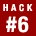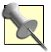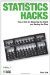# Hack 6. Measure PreciselyClassical test theory provides a nice analysis of the components that combine to produce a score on any test. A useful implication of the theory is that the level of precision for test scores can be estimated and reported.

A good educational or psychological test produces scores that are valid and reliable. Validity is the extent to which the score on a test represents the level of whatever trait one wishes to measure, and the extent to which the test is useful for its intended purpose. To demonstrate validity, you must present evidence and theory to support that the interpretations of the test scores are correct.

Reliability is the extent to which a test consistently produces the same score upon repeated measures of the same person. Demonstrating reliability is a matter of collecting data that represent repeated measures and analyzing them statistically.

#### Classical Test Theory

Classical test theory, or reliability theory, examines the concept of a test score. Think of the observed score (the score you got) on a test you took sometime. Classical test theory defines that score as being made up of two parts and presents this theoretical equation:

Observed Score = True Score + Error Score

This equation is made up of the following elements:

Observed score

The actual reported score you got on a test. This is typically equal to the number of items answered correctly or, more generally, the number of points earned on the test.

True score

The score you should have gotten. This is not the score you deserve, though, or the score that would be the most valid. True score is defined as the average score you would get if you took the same test an infinite number of times. Notice this definition means that true scores represent only average performance and might or might not reflect the trait that the test is designed to measure. In other words, a test might produce true scores, but not produce valid scores.

Error Score

Under this theory, it is assumed that performance on any test is subject to random error. You might guess and get a question correct on a social studies quiz when you don't really know the answer. In this case, the random error helps you.Notice this is still a measurement "error," even though it increased your score.

You might have cooked a bad egg for breakfast and, consequently, not even notice the last set of questions on an employment exam. Here, the random error hurt you. The errors are considered random, because they are not systematic, and they are unrelated to the trait that the test hopes to measure. The errors are considered errors because they change your score from your true score.

Over many testing times, these random errors should sometimes increase your score and sometimes decrease it, but across testing situations, the error should even out. Under classical test theory, reliability [Hack #31] is the extent to which test scores randomly fluctuate from occasion to occasion. A number representing reliability is often calculated by looking at the correlations among the items on the test. This index ranges from 0.0 to 1.0, with 1.0 representing a set of scores with no random error at all. The closer the index is to 1.0, the less the scores fluctuate randomly.

#### Standard Error of Measurement

Even though random errors should cancel each other out across testing situations, less than perfect reliability is a concern because, of course, decisions are almost always made based on scores from a single test administration. It doesn't do you any good to know that in the long run, your performance would reflect your true score if, for example, you just bombed your SAT test because the person next to you wore distracting cologne.

Measurement experts have developed a formula that computes a range of scores in which your true level of performance lies. The formula makes use of a value called the standard error of measurement. In a population of test scores, the standard error of measurement is the average distance of each person's observed score from that person's true score. It is estimated using information about the reliability of the test and the amount of variability in the group of observed scores as reflected by the standard deviation of those scores [Hack #2].

The formula for the standard error of measurement is:

Here is an example of how to use this formula. The Graduate Record Exam (GRE) tests provide scores required by many graduate schools to help in making admission decisions. Scores on the GRE Verbal Reasoning test range from 200 to 800, with a mean of about 500 (it's actually a little less than that in recent years) and a standard deviation of 100.

Reliability estimates for scores from this test are typically around .92, which indicates very high reliability. If you receive a score of 520 when you take this exam, congratulations, you performed higher than average. 520 was your observed score, though, and your performance was subject to random error. How close is 520 to your true score? Using the standard error of measurement formula, our calculations look like this:

1. 1 - .92 = .08

2. The square root of .08 is .28

3. 100x.28 = 28

The standard error of measurement for the GRE is about 28 points, so your score of 520 is most likely within 28 points of what you would score on average if you took the test many times.

#### Building Confidence Intervals

What does it mean to say that an observed score is most likely within one standard error of measurement of the true score? It is accepted by measurement statisticians that 68 percent of the time, an observed score will be within one standard error of measurement of the true score. Applied statisticians like to be more than 68 percent sure, however, and usually prefer to report a range of scores around the observed score that will contain the true score 95 percent of the time.

To be 95 percent sure that one is reporting a range of scores that contain an individual's true score, one should report a range constructed by adding and subtracting about two standard errors of measurement. Figure 1-1 shows what confidence intervals around a score of 520 on the GRE Verbal test look like.

##### Figure 1-1. Confidence intervals for a GRE score of 520#### Why It Works

The procedure for building confidence intervals using the standard error of measurement is based on the assumptions that errors (or error scores) are random, and that these random errors are normally distributed. The normal curve [Hack #25] shows up here as it does all over the world of human characteristics, and its shape is well known and precisely defined. This precision allows for the calculation of precise confidence intervals.

The standard error of measurement is a standard deviation. In this case, it is the standard deviation of error scores around the true score. Under the normal curve, 68 percent of values are within one standard deviation of the mean, and 95 percent of scores are within about two standard deviations (more exactly, 1.96 standard deviations). It is this known set of probabilities that allows measurement folks to talk about 95 percent or 68 percent confidence.

#### What It Means

How is knowing the 95 percent confidence interval for a test score helpful? If you are the person who is requiring the test and using it to make a decision, you can judge whether the test taker is likely to be within reach of the level of performance you have set as your standard of success.

If you are the person who took the test, then you can be pretty sure that your true score is within a certain range. This might encourage you to take the test again with some reasonable expectation of how much better you are likely to do by chance alone. With your score of 520 on the GRE, you can be 95 percent sure that if you take the test again right away, your new score could be as high as 576. Of course, it could drop and be as low as 464 the next time, too.Statistics Hacks: Tips & Tools for Measuring the World and Beating the Odds
ISBN: 0596101643
EAN: 2147483647
Year: 2004
Pages: 114
Authors: Bruce Frey

Similar book on Amazon